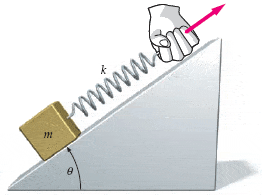# Potential Energy, Springs, and Friction

cowmoo32

## Homework Statement

A block of mass m rests on a plane inclined at an angle θ with the horizontal. A spring with force constant k is attached to the block. The coefficient of static friction between the block and plane is μs. The spring is pulled upward along the plane very slowly.

What is the extension of the spring the instant the block begins to move. (Use any variable or symbol stated above along with the following as necessary: g.)F = ma
F = -kx

## The Attempt at a Solution

I figured I can sum up forces in X and Y, with X being in the direction of the plane, and solve for x, but it's coming back as incorrect. I tried reversing the signs in case I missed a negative as well. The only other option I can see is summing the forces in the X direction equal to zero which would remove the ma term, but the system could be in equilibrium for any value of X and the block still not move. My thoughts are that setting Fx=ma finds x the instant it moves. I only have one submission left so I wanted to check before I go any farther.

ƩFy=FN-mgcos(θ)=0

FN=mgcos(θ)

ƩFx=-FNμs-mgsin(θ)+kx=ma

Substitute FN
-mgcos(θ)μs-mgsin(θ)+kx=ma

Solving for x
(-mgcos(θ)μs-mgsin(θ)-ma)/k=x

Staff Emeritus
Gold Member
At the instant the block begins to move, the spring force pulling up the incline has *only just barely* exceeded the weight and the static frictional force that are pulling down the incline. So you can set sum of the forces = 0 in the horizontal direction to get the critical value of x above which the thing will begin moving.

In other words, do ƩFx = 0, NOT ƩFx = ma, because what the heck would you use for the value of a?

cowmoo32
Gotcha, thanks. And just to be clear, is the spring -kx or kx since it's in the positive x direction? Negatives kill me.

Edit: Never mind. If I make it negative, I get a negative value for x, which makes no sense given my origin.

Last edited:
Staff Emeritus